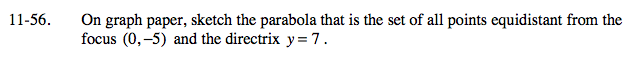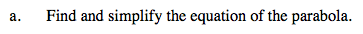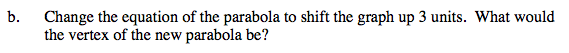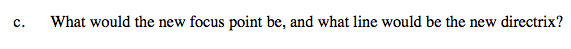Home > A2C > Chapter 11 > Lesson 11.2.2 > Problem11-56

11-56.
1. On graph paper, sketch the parabola that is the set of all points equidistant from the focus (0, −5) and the directrix y = 7. Homework Help ✎

1. Find and simplify the equation of the parabola.

2. Change the equation of the parabola to shift the graph up 3 units. What would the vertex of the new parabola be?

3. What would the new focus point be, and what line would be the new directrix?Now simplify.What operation do you perform on the equation to shift the graph up 3 units?When the graph is shifted up 3 units, what happens to the focus and directrix?